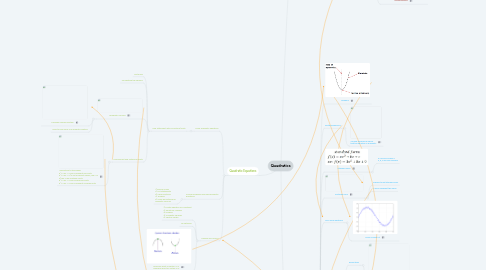Haranyaa Vigneswaran
Get Started. It's FreeQuadratics### 1.1. Multiplying Polynomials and Binomials

1.1.1. Polynomials

1.1.2. Binomials

### 1.2. Special Products

1.2.1. Perfect Square Trinomial

1.2.2. Difference of Squares

### 1.3. Common Factors

1.3.1. Group Factoring

1.3.1.1. May use multiple techniques

1.3.2. Greatest Common Factor to find two or more terms

1.3.3. Difference of Squares to find two terms

### 1.4. Factor form ax^2 + bx + c

1.4.1. Inspection

1.4.2. Decomposition

### 2.2. Finite Differences

2.2.1. Second Difference means that the equation is quadratic

### 2.3. Standard Form

2.3.1. a CANNOT equal 0 a, b, c are real numbers

### 2.4. Factored Form

2.4.1. Expand to get standard form

2.4.2. r and s represent the zeros

### 2.5. Non-linear Relations

2.5.1. Curve of Best Fit

### 2.6. Transformations

2.6.1. Reflections

2.6.1.1. a<0

2.6.2. Vertical Stretch

2.6.2.1. a>1 or a<-1

2.6.3. Vertical Compression

2.6.3.1. 0<a<1 or -1<a<0

2.6.4. Translations

2.6.4.1. Units Right: h>0

2.6.4.2. Units Left: h<0

2.6.4.3. Units Up: b>0

2.6.4.4. Units Down: b<0

### 2.7. Vertex Form

2.7.1. Complete Squares from Standard form

2.7.2. Opens up: a>0 Opens Down: a<0 X Values/Domain: D{x|xεR} Y Values/Range: if a>0, then y ≥ b if a<0, then y ≤ b

### 2.8. Zeros

2.8.1. b^2-4ac > 0 has 2 zeros

2.8.2. b^2-4ac = 0 has 1 zero

2.8.3. b^2-4ac < 0 has no zeros

3.1.1. Find Statement with 2 solutions/roots

3.1.1.1. Factoring

3.1.1.2. Completing the squares

3.1.1.3.1. Complex Number System

3.1.1.3.2. Used to find zeros of a quadratic relation

3.1.1.4. Discriminant and Nature of Roots

3.1.1.4.1. *correction to the image: b^2-4ac > 0 has 2 different real roots b^2-4ac > 0 (if not a perfect square, like 2 or 3) has 2 real irrational roots b^2-4ac = 0 has 2 equal real roots b^2-4ac < 0 has 2 different complex roots

### 3.2. Solving Problems involving Quadratic Equations

3.2.1. 1) Build a Model 2) Let Statements 3) Clear Fractions 4) Simplify 5) Solve by factoring or quadratic formula

### 3.3. Maxima and Minima

3.3.1. 1) Create Equation of Constraint 2) Isolate for Variable 3) Simplify 4) Complete Squares 5) Identify vertex

3.3.2. To Optimize

3.3.3. Minimum point of vertex: a>0 Maximum point of vertex: a<0